Relative Motion | Relative Velocity in One & Two Dimensions

Relative motion typically refers to, either the, relative position of one object as measured from the other object, or, relative velocity of one object with respect to another.

So, we will start our discussion with relative position and you will find two practice problems on relative position to help improve your understanding

Afterwards we will discuss relative velocity of one object with respect to another in one dimension followed by relative velocity in two dimensions where we will review rain man problem, the swimmer in river problem, and projectile problem where in projectile is projected from a moving platform at some relative velocity and projection angle and we will need to determine the initial velocity and projection angle with respect to the ground. Note that in all these 3 types of problems, we are either interested in establishing relative velocity vector in 2 dimensions or the velocity vector with respect to the ground.

Afterwards we will review the ways to a) determine minimum distance between two objects moving in two dimensions and b) determine time to collision for two objects moving in two dimensions.

So starting with relative position,

Relative Position

For two particles, $A$ and $B$, moving along one dimension, say in the vertical direction, relative position of $A$ with respect to $B$ at some time $t$ would simply be $y_A(t) – y_B(t)$ and if we were to write the relative position of $B$ with respect to $A$ it would be $y_B(t) – y_A(t)$

Now, let’s define the relative position of $A$ with respect to $B$ (or vice versa) in two or three dimensions.

So, if the position vectors of $A$ and $B$ at some time $t$ are $\overrightarrow{r}_A(t)$ and $\overrightarrow{r}_B(t)$, then relative position vector of $A$ with respect to $B$ would be $\overrightarrow{r}_A(t) – \overrightarrow{r}_B(t)$ which we can write in the unit vector notation as $(x_A – x_B) \hat{i} +$ $(y_A – y_B) \hat{i} +$ $(z_A – z_B) \hat{k}$

It’s that simple and straightforward. Now let’s solve two problems that will challenge our problem solving skills. (Feel free to refer to solution videos to improve your understanding)

Q1. Two stones are thrown up simultaneously from the edge of a cliff $240 \ m$ high with initial speed of $10 \ m/s$ and $40 \ m/s$ respectively. Plot the graph representing the time variation of relative position of the second stone with respect to the first? (Assume stones do not rebound after hitting the ground & neglect air resistance, $g = 10 \ m/s^2$)

Correct graph is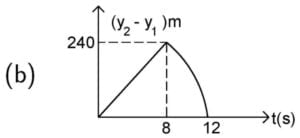Play Video

Q2. A body is at rest at $x = 0$. At $t = 0$, it starts moving in the positive $x$-direction with a constant acceleration. At the same instant, another body passes through $x = 0$ moving in the positive $x$-direction with a constant speed. The position of the first body is given by $x_{1}(t)$ after time $t$ and that of the second body by $x_{2}(t)$ after the same time interval. Plot the graph which correctly describes $(x_{2} – x_{1})$ as a function of time?

Correct graph is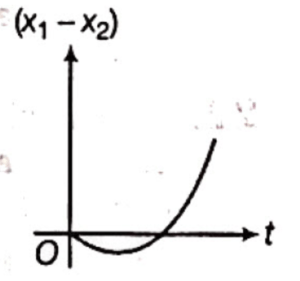Play Video

Relative Velocity | One Dimensional Motion

Relative velocity of $A$ with respect to $B$ is the rate at which relative position of $A$ with respect to $B$ is changing with time.

If this sounded technical, take a look at the following two animations with both the objects moving in the same direction in first animation and moving in opposite directions in the second animation.

Note that $A$ has only moved by a distance $x_{AB}$ with respect to $B$. So, $B$ would observe $A$ to be moving at a speed of $v_{AB}$

Now, if you were given $v_{AB}$ and $v_{B}$, can you find $v_{A}$? (it will be $v_{B} + v_{AB}$)

Here $A$ and $B$ are moving in opposite directions, so $B$ would observe $A$ to be moving at a higher speed compared to speed of $A$ with respect to an observer on the ground.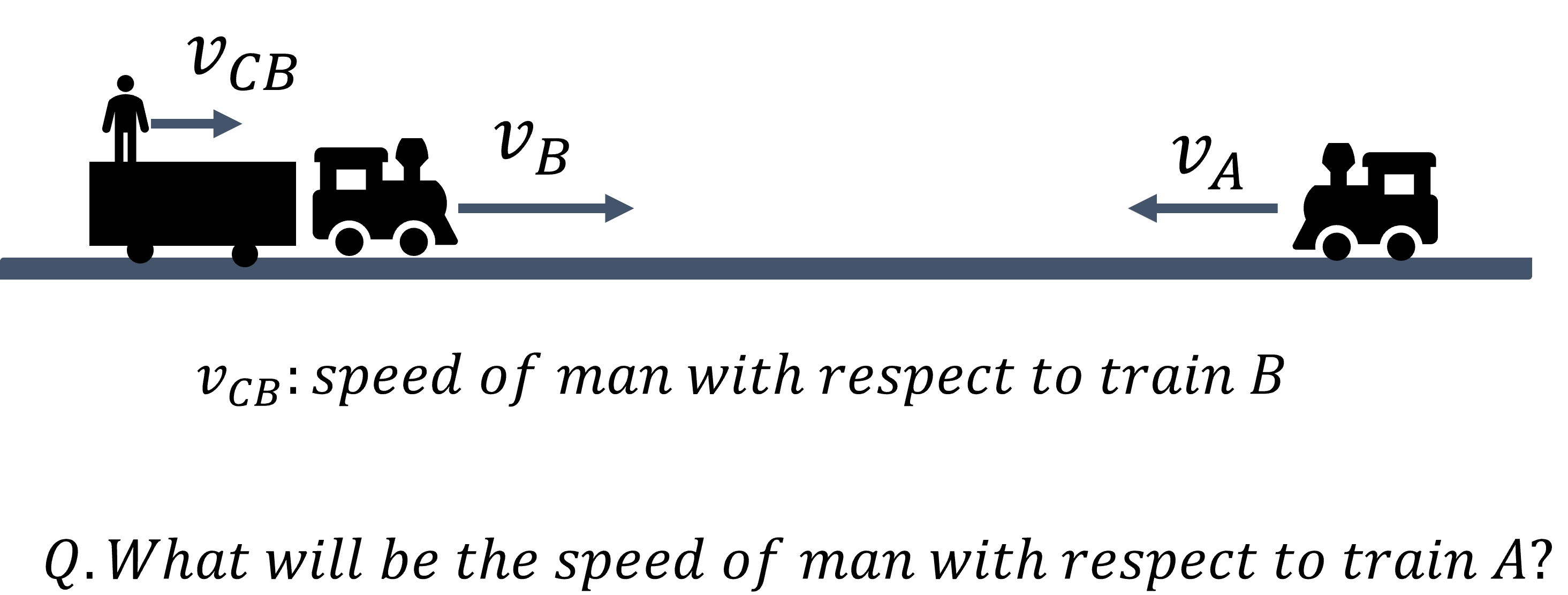Let’s test our understanding of relative velocity in one dimension.

Here you have been given velocity of man (C) with respect to train B. And you have also been given speed of train B and speed of another train A with respect to ground. So, can you determine the speed of man with respect to train A?

Let’s solve a practice problem on the same. If you have any difficulty, take a look at the solution video

Q3. Train A and train B are running on parallel tracks in the opposite directions with speeds of 36 km/hour and 72 km/hour, respectively. A person is walking in train A in the direction opposite to its motion with a speed of 1.8 km/hour. Speed (in ms$^–1$) of this person as observed from train B will be close to : (take the distance between the tracks as negligible)

(a) 29.5 ms$^{-1}$               (b) 30.5 ms$^{-1}$

(c) 31.5 ms$^{-1}$               (d) 28.5 ms$^{-1}$

Play Video

Time to Meet / Collide | One Dimensional Motion

Now let’s say that you have been given that, two objects, A and B, are moving towards each other at speeds $v_A$ and $v_B$. So, after how much time will they collide with each other if they were at a distance $d$ from each other at time $t=0$.

Well, let’s try and answer it using relative velocity.

So, relative velocity of say A with respect to B will be $v_A + v_B$ in this case and at $t=0$ observer on B will report that A is at a distance $d$ from B and moving towards it at a speed of $v_A + v_B$.

So time taken for A to reach B would be $=\cfrac{d}{v_A + v_B}$

With that, let’s move to two dimensional scenarios and first learn to establish relative velocity in two dimensions following which we will examine problems where we will use relative velocity to determine minimum distance between two objects moving at constant velocities in 2 dimensions or time for two objects to collide in 2 dimensions.

Relative Velocity | Two Dimensional Motion | Rain Man ProblemIn vector form relative velocity of $A$ with respect to $B$ would always be $\overrightarrow{v}_A – \overrightarrow{v}_B$ where $\overrightarrow{v}_A$ and $\overrightarrow{v}_B$ are the velocity vectors of $A$ and $B$ with respect to ground.

Here understanding of graphical representation of vector addition and subtraction would help. So make sure that you revisit your concepts on vectors

Now let’s take a look at a sample practice problem to help better understand the concept of relative velocity in two dimensions

Q4. On a rainy day, a man is running on a hilly terrain in such a way that he is always finding the rain drops hitting him vertically. It is assumed that rainfall is uniform in the whole terrain from $O$ to $E$. When the man was at rest on stretch $OA$, he found the rainfall at an angle of $30^{\circ}$ with the vertical.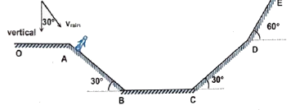Determine the following

(a) The magnitude of velocity of rain observed on stretch $AB$ to the magnitude of velocity of man on stretch $BC$.

(b) The magnitude of velocity of rain observed on stretch $BC$ to the magnitude of actual velocity of rain.

(c) The magnitude of velocity of rain observed on stretch $DE$ to the magnitude of velocity of rain observed on stretch $CD$.

(d) The magnitude of velocity of man on stretch $DE$ to the magnitude of velocity of man on stretch $CD$

(a) $2/\sqrt{3}$

(b) $\sqrt{3}/2$

(c) $3/2$

(d) $\sqrt{3}$

Play Video

Relative Velocity | Two Dimensional Motion | Swimmer in River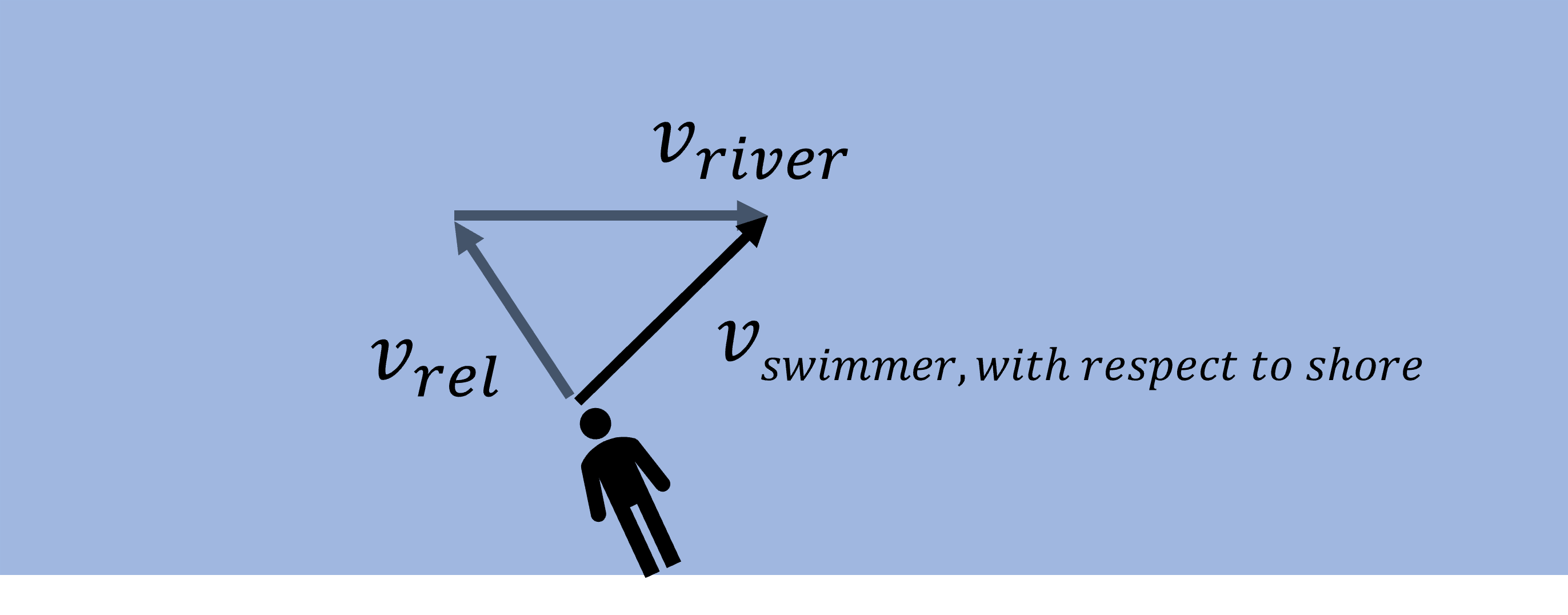It is similar to rain man problem and you are typically given the speed of swimmer with respect to still water and you are also given the speed of the river.

Here you will typically be asked to determine

a) Minimum time for the swimmer to cross the river – This will happen when the relative velocity of swimmer with respect to water is perpendicular to the shore because time to cross is determined by the component of velocity perpendicular to the shore and it will be $=\cfrac{d}{v_{rel}}$, where $d$ is the width of the river

b) Time to cross the river if the swimmer has to land on the other shore just opposite to his launch point. Here swimmer will have to take off at an angle $\theta$ from the perpendicular to shore such that $\overrightarrow{v}_{rel} + \overrightarrow{v}_{river}$, the velocity vector, as observed by an observer on the ground, is perpendicular to the shore

c) Landing point of the swimmer if he/she swims perpendicular to the flow of the river. Use $\overrightarrow{v}_{rel} + \overrightarrow{v}_{river}$ to determine the velocity vector with respect to the ground and the angle this velocity vector makes with the shore. You can then easily determine the landing point

Now let’s take a look at a practice problem that will test your application of concepts.!

Q5. A river is flowing with the velocity of $2\sqrt{3} \ m/s$. A boat has to move from $A$ to $B$. Find the minimum velocity of boat in still water.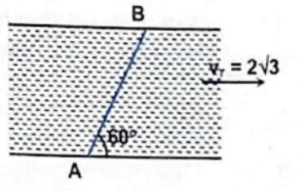Correct answer is $3 \ m/s$.

Relative Velocity | Two Dimensional Motion | Projectile launched from a moving platform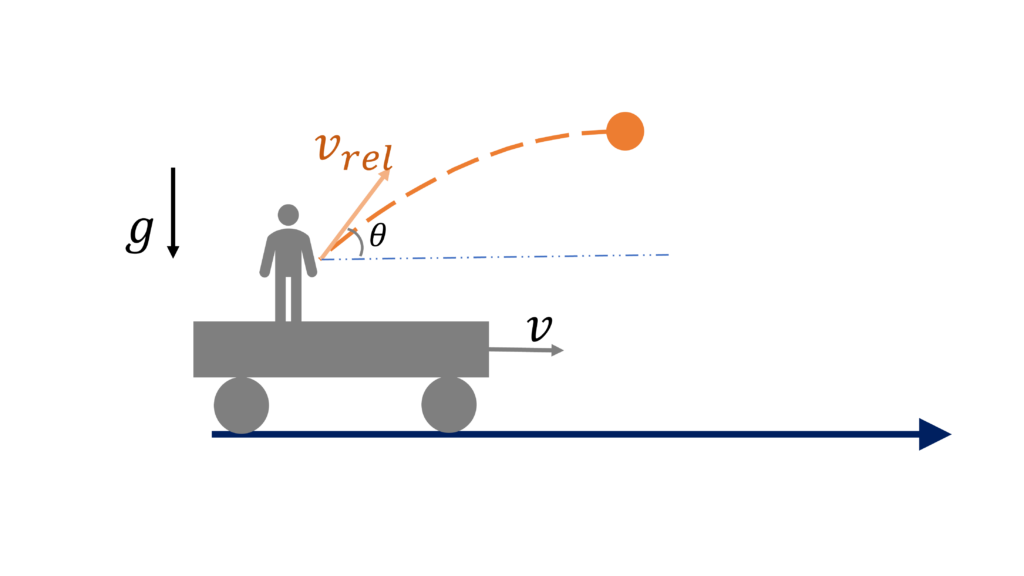Given that the platform is moving with speed $v$ towards right and the projectile is launched at a relative speed $u$ at an angle $\theta$ with the horizontal, as seen by an observer on the platform, can you determine the launch speed and projection angle with respect to an observer on the ground

Well the velocity vector with respect to ground will be $(v + u \cos \theta) \hat{i} + u \sin \theta \hat{j}$

So, speed with respect to ground would be $\sqrt{(v + u \cos \theta)^2 + u^2 \sin^2 \theta}$ and the projection angle as seen by observer on the ground would be $\tan^{-1} \cfrac{u \sin \theta}{v + u \cos \theta}$

Now let’s solve a practice problem on the same

Q6. A train is moving along a straight line with a constant acceleration $a$. A boy standing in the train throws a ball forward with a speed of $10 \ m/s$, at an angle of $60^{\circ}$ to the horizontal. The boy has to move forward by $1.15 \ m$ inside the train to catch the ball back at the initial height. The acceleration of the train, in $m/s^2$, is :

(a) $2 \ m/s^2$             (b) $5 \ m/s^2$

(c) $6 \ m/s^2$             (d) $11 \ m/s^2$

Play Video

Minimum distance between two particles moving at constant velocities (2D Motion)

Here two objects are moving in straight lines at given speeds in two dimensions and we need to determine the minimum distance between the two or find the time taken for them to get closest to each other, given their initial positions.

Hope the above animation has given you a clue on the same but let’s take a look at a sample practice problem to further our understanding.

Q7. Ship A is sailing towards north-east with velocity $\overrightarrow{v} = 30 \hat{i} + 50 \hat{j}$ km/hr where $\hat{i}$ point east and $\hat{j},$ north . Ship B is at a distance of 80 km east and 150 km north of Ship A and is sailing towards west at 10 km/hr . A will be at minimum distance from B in :

(a) $4.2$ hrs                      (b) $2.2$ hrs

(c) $3.2$ hrs                     (d) $2.6$ hrs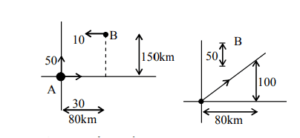$\hat{V}_{AB} = 40 \hat{i} + 50\hat{j}$
$\overrightarrow{r}_{BA} = 80 \hat{i} + 150 \hat{j}$
$t_{min} = \cfrac{|\overrightarrow{V}_{AB} \bullet \overrightarrow{r}_{BA}|}{|\overrightarrow{V}_{AB}|^2}$ = 2.6 hrs

Time to meet / collide | Two Dimensional Motion | Particles under constant acceleration

Q8. A particle is projected from ground at an angle $60^{\circ}$ with horizontal with a speed of $10\sqrt{3} \ m/s$ from point $A$ as shown. At the same time the sufficient long wedge is made to move with constant velocity of $10\sqrt{3}$ towards right as shown in figure. The time in second after which particle will hit the wedge will be. ($g = 10 \ m/s^2$)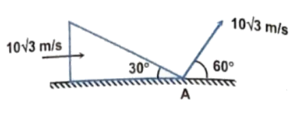(a) $1 \ sec$            (b) $2 \ sec$

(c) $3 \ sec$           (d) it will never collide on the wedge